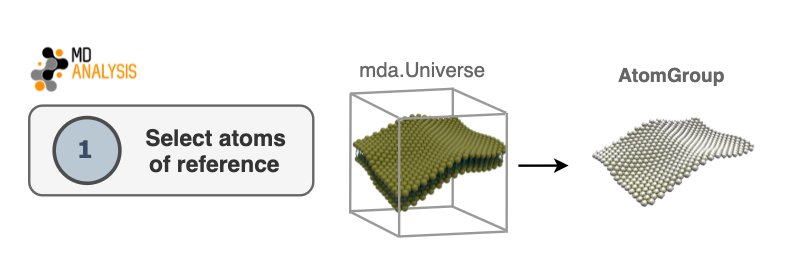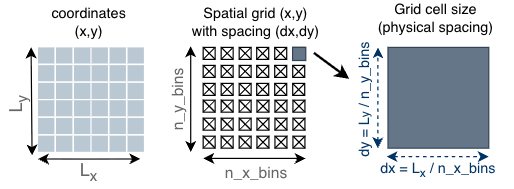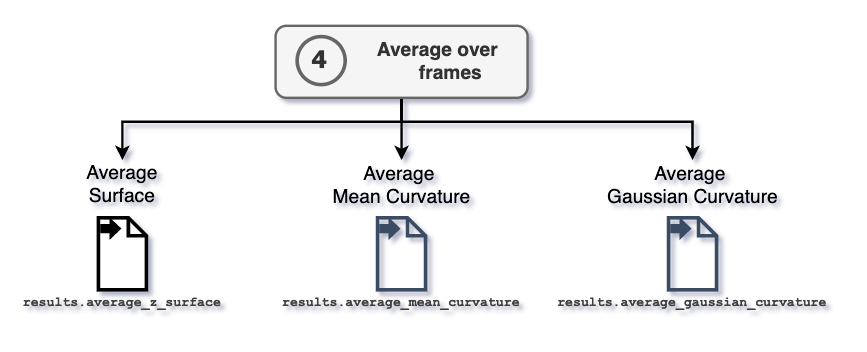# Algorithm

## Overview

MembraneCurvature calculates mean and Gaussian curvature of surfaces derived from atoms of reference in 4 steps:

1. Select atoms of reference

2. Set grid

3. Derive surface and calculate curvature

4. Average over frames

A summary of the algorithm used in MembraneCurvature is shown in the following diagram:## 1. Select atoms of reference

The first step in the algorithm consists of selecting atoms that will be used as a reference to derive a surface. This selection will be contained in an `AtomGroup`. Typically in biological membranes, lipid headgroups are the most common elements to use as an AtomGroup of reference.## 2. Set grid

The dimensions of the grid are determined by the size of the simulation box contained in the `Universe`. The grid comprises `n_x_bins` x `n_y_bins` number of bins.For every atom in the `AtomGroup` of reference, MembraneCurvature assigns an index in the grid, according to their respective x and y coordinates. i.e. `(x, y) ↦ [l, m]`.

Note

Unless the user provides a different input, MembraneCurvature will determine the dimensions of the grid based on the size of the box on the first frame via `dimensions`.

```grid_dimension_x = (0, universe.dimensions)
grid_dimension_y = (0, universe.dimensions)
```

Once the grid is populated according to the coordinates of the atoms in the AtomGroup of reference, the associated z coordinate of each atom in the AtomGroup is stored in an array assigned to each `[l, m]` index.

## 3. Derive surface and calculate curvature

Once the surface formed by the atoms of reference is derived, values of mean (H) and Gaussian (K) curvature are calculated according to their respective equations.

For every frame of the trajectory, the surface derived from the `AtomGroup` is calculated and stored in `z_surface`. Similarly, the calculation of mean and Gaussian curvature is performed in every frame and stored in `MembraneCurvature.results.mean_curvature` and `MembraneCurvature.results.gaussian_curvature`, respectively.## 4. Average over frames

The attributes `MembraneCurvature.results.average_mean` and `MembraneCurvature.results.average_gaussian` contain the computed values of mean and Gaussian curvature averaged over all the `n_frames` in the trajectory.

After performing the average over frames, the information of average surface, mean, and Gaussian curvature are stored in the `MembraneCurvature.results.average_z_surface`, `MembraneCurvature.results.average_mean`, and `MembraneCurvature.results.average_gaussian` arrays, respectively. Each array has shape `(n_x_bins, n_y_bins)`.